Home » Our Blog » How to subtract in Excel

# How to subtract in Excel

It's simple when you're shown how, but read to the end to make sure you understand what happens when you multiply and subtract, or divide and subtract in the same formula.

This is a tricky one, so please don’t skip over it!

## ​How to subtract in Excel

Let’s look at writing a subtraction formula in Excel.

In the example below we are going to take away or subtract the money that we've spent from our budget, to calculate the difference. The result will be held in cell C4.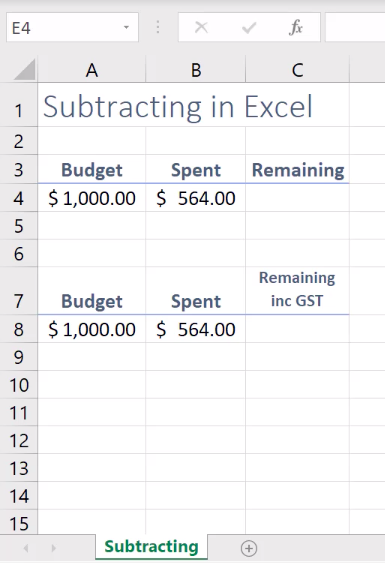## Step 1: select the cell that will hold the subtraction formula and then press equals

When you're writing a formula within Excel, whether you're doing multiplication, addition, subtraction, division, you always need to click on the cell where you will be typing the formula first.

In the example above this would be cell C4.

Once you’ve done this you need to tell Excel you're about to do a calculation.

We do this by pressing our equals key (=) on the keyboard.

When you press equals, you're now saying to Excel, "Hey, I'm about to go and do a calculation" and Excel sits up and takes notice.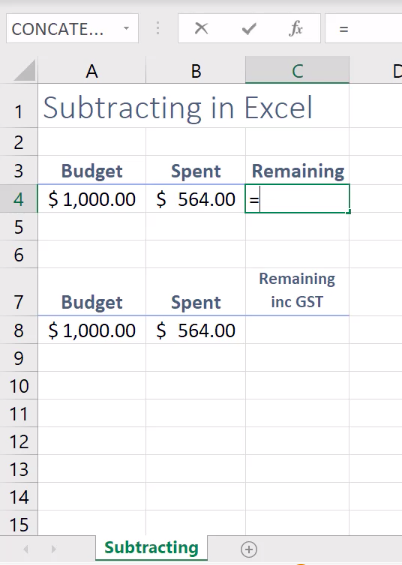## ​Step 2: type the subtraction formula

### ​Subtracting using real numbers

I watch a lot of people write subtraction formulas using real numbers.

Although Excel will always give you the correct answer when you subtract this way, this isn’t always the best way to subtract in Excel

Let’s look at this method first and then I’ll explain why this isn’t always the best method.

In the example below 1000 has been typed after the equals sign.

The subtraction or minus sign (-) has then been typed. You can find the subtraction symbol on your numeric keyboard, or just to the left of your equals key on the keyboard.

Then 564 has been typedThe formula is =1000-564.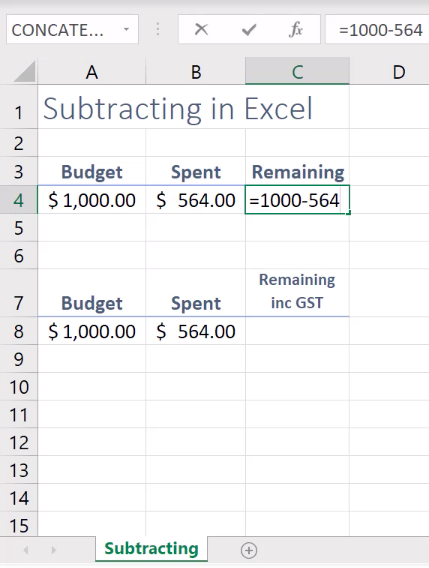We perform the calculation by pressing the Enter key on the keyboard.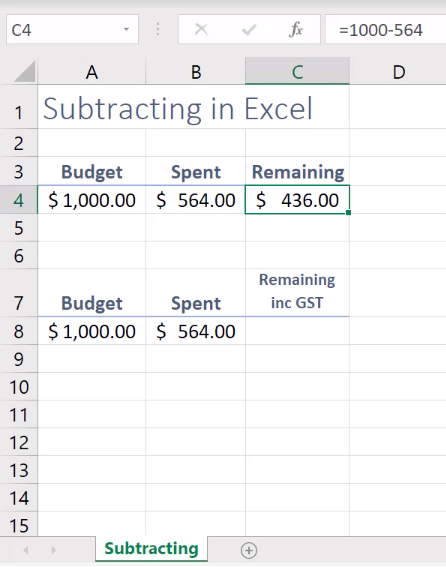Excel will perform the calculation and give us the correct answer. You will be able to see the result down on the grid part of your worksheet and the formula up in the Formula Bar.However, what if we now realised our budget was \$1200, it wasn't \$1000, and we now need to update the value in cell A4 to \$1200.

Wouldn't it be great if when we updated the value in A4, the Remaining value in C4 immediately updated to reflect the difference?

So, let’s look at creating a subtraction formula in Excel using cell references instead of real numbers.

### ​Subtraction formulas using cell references

In the example below I’m now referencing the cells holding the information.

The formula is =A4-B4, meaning you are now asking Excel to subtract the value held in cell B4 from the value in A4.

The beauty about this, is when you reference cells, instead of the real numbers, should you update a value in a cell used in a formula, the result will automatically update.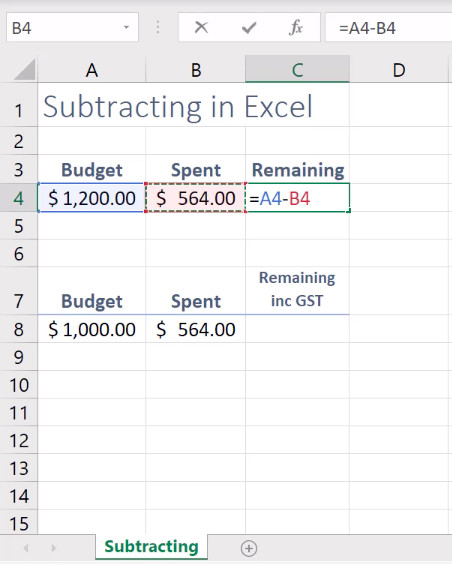Tip: if you wanted to, you could actually type =A4-B4 into the cell. You don't need uppercase A, uppercase B. A quicker way to place cell references into a formula is to press = and then just click on to the cell you require and the cell reference will be added to your formula (you can see me doing this in the video at the top of this blog)

## ​Using brackets in subtraction formulas in Excel

It's really important that you understand what can happen when you're creating formulas with more than just the subtraction or minus sign. Let’s say you needed to create a formula that included a subtraction AND a multiplication.

There is a key element to these formulas that you need to know to avoid incorrect calculations.

In the example below I'm using an asterisk for multiplication and including a multiplication into the formula to include GST in my calculation.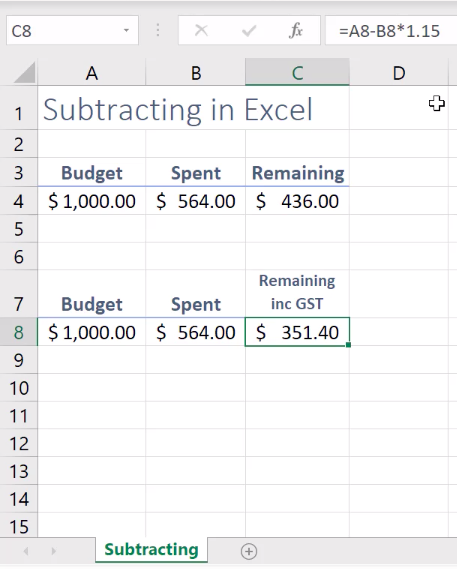The problem is I've ended up with a figure that is actually less than what we had originally in C4.

So, let me explain what's happened.

When you have more than just the subtraction symbol in a formula, you need to look at that formula and think, "What part of this formula needs to be calculated first?"

There is a rule behind this called the BEDMAS rule, also known as the BODMAS or PEDMAS rule.

A simple overview of this rule applied in Excel is this. If you are looking at a formula, and a certain part of that formula needs to be calculated first, you put brackets around it.

Without the brackets, Excel is actually whipping onto B8 and multiplying that by 1.15 first, then it's deducting the A8.

Sound complicated?

Don’t be concerned.

All you need to remember is that when you have more than one operator, for example, multiplication sign, a plus sign, or a division sign, included with your subtraction sign in a formula, make sure that you bracket the part of the formula that needs to be calculated first.

That's it. That's all you have to remember.

Take a look at the formula and the result now that we have included brackets.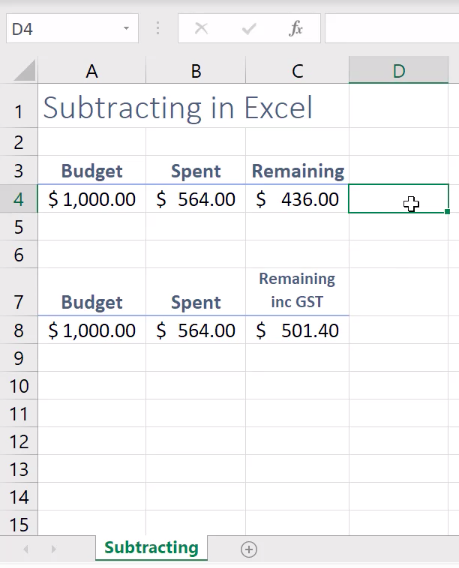The brackets around the A8 minus the B8, means that Excel will calculate this part of the formula first

Excel will then take the result if this calculation, and then carry on and multiply by 1.15.

I really hope you're feeling confident about subtracting in Excel now.

Just remember to use cell references instead of real numbers if you're going to update the cells regularly, and don't forget your brackets.

## Divide, multiply and addition formulas in Excel

Use the same steps as above to create division, multiplication and addition formulas in Excel.

The table below shows the standard operator symbols that are used in Excel for these calculations.

You can find these symbols on your keyboard and numeric pad (if your keyboard has one 😊).

For example, =B4/A4 will divide cell B4 into A4, =B4+C4 would add the two cells together and =C4*1.15 (which you have seen already) would multiply C4 by 1.15.

TO DO THIS...

USE THIS SYMBOL...

Divide

/ (forward slash)

Multiply

* (asterisk a.k.a. 'star')

+ (plus sign)Sharyn is an expert trainer.  She became the first certified Microsoft® MOUS Authorised Instructor in New Zealand.

She is endorsed by Microsoft® as a qualified Microsoft® Office Specialist and has more than 20 years of experience in the training industry, developing and delivering technology training workshops. Her approach to taking the “techie-speak” out of technology training has placed her as a preferred supplier to many of New Zealand’s leading organisations.

# If you enjoyed this you may also enjoy...

## How to unhide columns in Excel

Need a hand to unhide columns in Excel?   There are different methods or unhiding available to you, depending on the version of Excel you are using.  I’ll take you through the method that will work on any version.

## Best Excel Keyboard Shortcuts for Beginners | Tips and Tricks

Learn the BEST Excel keyboard shortcuts. Perfect for Beginners and for those who want to save time and work smarter. It doesn’t matter if I’m delivering a Stage 1 or a Stage 3 Excel training course – when I’m demonstrating to the class I whip around the screen, format, select and modify and update using shortcut

## How to remove Excel formulas and keep the results

If you share Excel files outside of your organisation you’ll enjoy this post where I share with you how to remove Excel formulas and keep the results so that you can confidently send a file knowing you aren’t disclosing confidential information inside of your formulas.

## Move, Copy and Paste information in Excel

Learn how to move, copy and paste information in Excel. Once you have mastered Cut, Copy and Paste you will find yourself shifting data around easily and reusing copied data, saving you the need to recreate the same information again. All Windows applications have access to the Windows Clipboard. This is a special area used for

## Add colour, borders and alignment settings to Excel data

Learn how to add colour, borders and alignment settings to your Excel data to give your worksheet a more professional look and to make it easier for others to read.

## Add dollar signs, percentage signs and decimal places to Excel data

Formatting lets you change the appearance of data to control exactly how Excel will display the data on a worksheet. An example of formatting is to add dollar signs, percentage signs and decimal places to Excel data.